Valence-Shell Electron-Pair Repulsion Theory (VSEPR)

Predicting the Shapes of Molecules

There is no direct relationship between the formula of a compound and the shape of its molecules. The shapes of these molecules can be predicted from their Lewis structures, however, with a model developed about 30 years ago, known as the valence-shell electron-pair repulsion (VSEPR) theory.

The VSEPR theory assumes that each atom in a molecule will achieve a geometry that minimizes the repulsion between electrons in the valence shell of that atom. The five compounds shown in the figure below can be used to demonstrate how the VSEPR theory can be applied to simple molecules.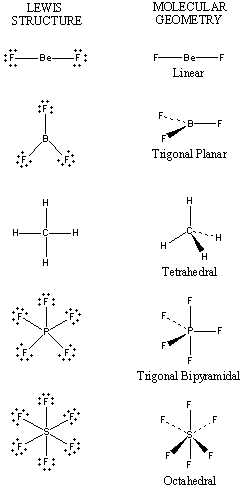There are only two places in the valence shell of the central atom in BeF2 where electrons can be found. Repulsion between these pairs of electrons can be minimized by arranging them so that they point in opposite directions. Thus, the VSEPR theory predicts that BeF2 should be a linear molecule, with a 180o angle between the two Be-F bonds.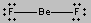There are three places on the central atom in boron trifluoride (BF3) where valence electrons can be found. Repulsion between these electrons can be minimized by arranging them toward the corners of an equilateral triangle. The VSEPR theory therefore predicts a trigonal planar geometry for the BF3 molecule, with a F-B-F bond angle of 120o.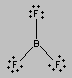BeF2 and BF3 are both two-dimensional molecules, in which the atoms lie in the same plane. If we place the same restriction on methane (CH4), we would get a square-planar geometry in which the H-C-H bond angle is 90o. If we let this system expand into three dimensions, however, we end up with a tetrahedral molecule in which the H-C-H bond angle is 109o28'.Repulsion between the five pairs of valence electrons on the phosphorus atom in PF5 can be minimized by distributing these electrons toward the corners of a trigonal bipyramid. Three of the positions in a trigonal bipyramid are labeled equatorial because they lie along the equator of the molecule. The other two are axial because they lie along an axis perpendicular to the equatorial plane. The angle between the three equatorial positions is 120o, while the angle between an axial and an equatorial position is 90o.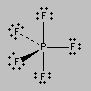There are six places on the central atom in SF6 where valence electrons can be found. The repulsion between these electrons can be minimized by distributing them toward the corners of an octahedron. The term octahedron literally means "eight sides," but it is the six corners, or vertices, that interest us. To imagine the geometry of an SF6 molecule, locate fluorine atoms on opposite sides of the sulfur atom along the X, Y, and Z axes of an XYZ coordinate system.Interactive tutorial on chemical bonds, molecular shapes, and molecular models by Dr. Anna Cavinato and Dr. David Camp, Eastern Oregon University,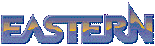Incorporating Double and Triple Bonds Into the VSEPR Theory

Compounds that contain double and triple bonds raise an important point: The geometry around an atom is determined by the number of places in the valence shell of an atom where electrons can be found, not the number of pairs of valence electrons. Consider the Lewis structures of carbon dioxide (CO2) and the carbonate (CO32-) ion, for example.There are four pairs of bonding electrons on the carbon atom in CO2, but only two places where these electrons can be found. (There are electrons in the C=O double bond on the left and electrons in the double bond on the right.) The force of repulsion between these electrons is minimized when the two C=O double bonds are placed on opposite sides of the carbon atom. The VSEPR theory therefore predicts that CO2 will be a linear molecule, just like BeF2, with a bond angle of 180o.

The Lewis structure of the carbonate ion also suggests a total of four pairs of valence electrons on the central atom. But these electrons are concentrated in three places: The two C-O single bonds and the C=O double bond. Repulsions between these electrons are minimized when the three oxygen atoms are arranged toward the corners of an equilateral triangle. The CO32- ion should therefore have a trigonal-planar geometry, just like BF3, with a 120o bond angle.The Role of Nonbonding Electrons in the VSEPR Theory

The valence electrons on the central atom in both NH3 and H2O should be distributed toward the corners of a tetrahedron, as shown in the figure below. Our goal, however, isn't predicting the distribution of valence electrons. It is to use this distribution of electrons to predict the shape of the molecule. Until now, the two have been the same. Once we include nonbonding electrons, that is no longer true.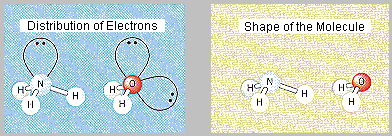The VSEPR theory predicts that the valence electrons on the central atoms in ammonia and water will point toward the corners of a tetrahedron. Because we can't locate the nonbonding electrons with any precision, this prediction can't be tested directly. But the results of the VSEPR theory can be used to predict the positions of the nuclei in these molecules, which can be tested experimentally. If we focus on the positions of the nuclei in ammonia, we predict that the NH3 molecule should have a shape best described as trigonal pyramidal, with the nitrogen at the top of the pyramid. Water, on the other hand, should have a shape that can be described as bent, or angular. Both of these predictions have been shown to be correct, which reinforces our faith in the VSEPR theory.

 Practive Problem 6:Use the Lewis structure of the ICl2+ ion shown in the figure below to predict the shape of this ion.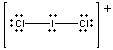Click here to check your answer to Practice Problem 6

 Practice Problem 7:Use the Lewis structure of the NO2 molecule shown in the figure below to predict the shape of this molecule.Click here to check your answer to Practice Problem 7

When we extend the VSEPR theory to molecules in which the electrons are distributed toward the corners of a trigonal bipyramid, we run into the question of whether nonbonding electrons should be placed in equatorial or axial positions. Experimentally we find that nonbonding electrons usually occupy equatorial positions in a trigonal bipyramid.

To understand why, we have to recognize that nonbonding electrons take up more space than bonding electrons. Nonbonding electrons need to be close to only one nucleus, and there is a considerable amount of space in which nonbonding electrons can reside and still be near the nucleus of the atom. Bonding electrons, however, must be simultaneously close to two nuclei, and only a small region of space between the nuclei satisfies this restriction.

Because they occupy more space, the force of repulsion between pairs of nonbonding electrons is relatively large. The force of repulsion between a pair of nonbonding electrons and a pair of bonding electrons is somewhat smaller, and the repulsion between pairs of bonding electrons is even smaller.

The figure below can help us understand why nonbonding electrons are placed in equatorial positions in a trigonal bipyramid.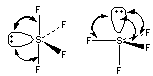If the nonbonding electrons in SF4 are placed in an axial position, they will be relatively close (90o) to three pairs of bonding electrons. But if the nonbonding electrons are placed in an equatorial position, they will be 90o away from only two pairs of bonding electrons. As a result, the repulsion between nonbonding and bonding electrons is minimized if the nonbonding electrons are placed in an equatorial position in SF4.

The results of applying the VSEPR theory to SF4, ClF3, and the I3- ion are shown in the figure below.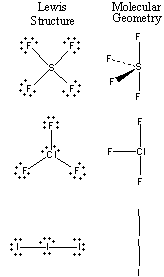When the nonbonding pair of electrons on the sulfur atom in SF4 is placed in an equatorial position, the molecule can be best described as having a see-saw or teeter-totter shape. Repulsion between valence electrons on the chlorine atom in ClF3 can be minimized by placing both pairs of nonbonding electrons in equatorial positions in a trigonal bipyramid. When this is done, we get a geometry that can be described as T-shaped. The Lewis structure of the triiodide (I3-) ion suggests a trigonal bipyramidal distribution of valence electrons on the central atom. When the three pairs of nonbonding electrons on this atom are placed in equatorial positions, we get a linear molecule.

Molecular geometries based on an octahedral distribution of valence electrons are easier to predict because the corners of an octahedron are all identical.